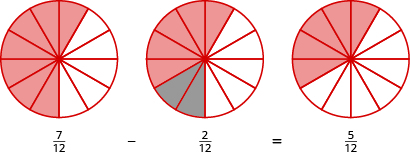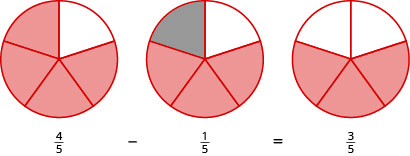## Subtracting Fractions With Common Denominators

### Learning Outcomes

• Use fraction circles to find the difference between two fractions with like denominators
• Subtract fractions with a like denominator without fraction circles
• Subtract fractions with like denominators that contain variables

## Model Fraction Subtraction

Subtracting two fractions with common denominators is much like adding fractions. Think of a pizza that was cut into $12$ slices. Suppose five pieces are eaten for dinner. This means that, after dinner, there are seven pieces (or ${\Large\frac{7}{12}}$ of the pizza) left in the box. If Leonardo eats $2$ of these remaining pieces (or ${\Large\frac{2}{12}}$ of the pizza), how much is left? There would be $5$ pieces left (or ${\Large\frac{5}{12}}$ of the pizza).

${\Large\frac{7}{12}}-{\Large\frac{2}{12}}={\Large\frac{5}{12}}$

Let’s use fraction circles to model the same example, ${\Large\frac{7}{12}}-{\Large\frac{2}{12}}$.

Start with seven ${\Large\frac{1}{12}}$ pieces. Take away two ${\Large\frac{1}{12}}$ pieces. How many twelfths are left?Again, we have five twelfths, ${\Large\frac{5}{12}}$.

### Example

Use fraction circles to find the difference: ${\Large\frac{4}{5}}-{\Large\frac{1}{5}}$

Solution:
Start with four ${\Large\frac{1}{5}}$ pieces. Take away one ${\Large\frac{1}{5}}$ piece. Count how many fifths are left. There are three ${\Large\frac{1}{5}}$ pieces left.## Subtract Fractions with a Common Denominator

We subtract fractions with a common denominator in much the same way as we add fractions with a common denominator.

### Fraction Subtraction

If $a,b,\text{ and }c$ are numbers where $c\ne 0$, then

${\Large\frac{a}{c}}-{\Large\frac{b}{c}}={\Large\frac{a-b}{c}}$

To subtract fractions with a common denominators, we subtract the numerators and place the difference over the common denominator.

### Example

Find the difference: ${\Large\frac{23}{24}}-{\Large\frac{14}{24}}$

### Try It

Watch the following video for more examples of subtracting fractions with like denominators.

### Example

Find the difference: ${\Large\frac{y}{6}}-{\Large\frac{1}{6}}$

### Example

Find the difference: ${\Large-\frac{10}{x}-\frac{4}{x}}$

### Try It

Now lets do an example that involves both addition and subtraction.

### Example

Simplify: ${\Large\frac{3}{8}}+\left(-{\Large\frac{5}{8}}\right)-{\Large\frac{1}{8}}$

### Try It

In the next video we show more examples of subtracting fractions with a common denominator.  Make note of the second example, it addresses a common mistake made by students when simplifying fractions with variables.